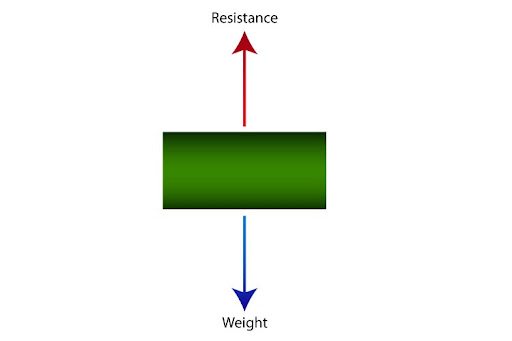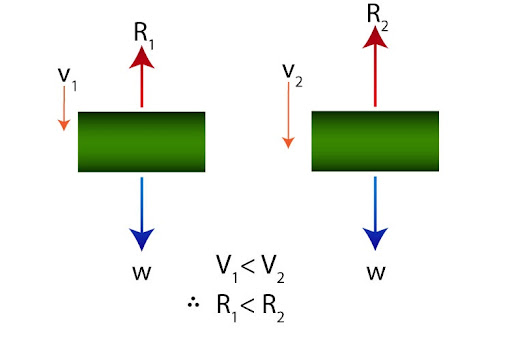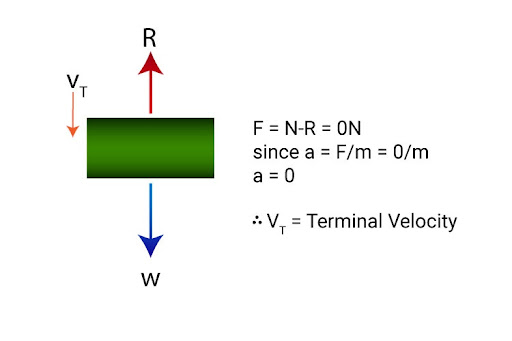# What is terminal velocity and what causes it?

Terminal velocity is defined as the constant - and maximum - velocity that a free-falling object attains as it falls through a particular medium (like air, water, or even honey!)

Now, constant velocity here implies that we’re talking about a specific scenario in which a falling object is moving with zero acceleration. And since F = ma, this means resultant force is also zero.

Keep note of our verbiage here: the resultant force acting on the object is zero, but that does not mean that there are no forces acting on it! In fact, there are precisely two forces acting on an object that falls freely: it’s weight and a resistive force offered by the medium, which by nature acts in the opposite direction.Weight in itself is dependent on the mass of the object (since W = mg), and so we expect that to remain unchanged as the object falls from rest due to its weight, but the resistive force acting on it will change. As the object picks up velocity, resistive force also keeps on increasing. Since resultant force F = W - R, then we can see F will steadily decrease, ultimately causing it’s acceleration to decrease too:Keep in mind that while the acceleration itself is decreasing, the velocity is steadily increasing throughout! Just to put it into perspective: the velocity is continuously increasing, but the change in velocity over each equal interval of time is decreasing. (an example: suppose the velocity of a free-falling object was increasing from 5 m/s to 10 m/s in the first 10 seconds, but in the next 10 seconds, it increases from 10 m/s to just 12 m/s - there’s still an appreciable increase, but a lesser one!)

There will eventually come a point when the resistive force becomes exactly equal to its weight - that is when the resultant force drops to zero, thereby leading to zero acceleration. Thus, the object attains terminal velocity.Now you may appreciate why we highlight this as the maximum velocity, because the object cannot accelerate any further than this!